Project tutorial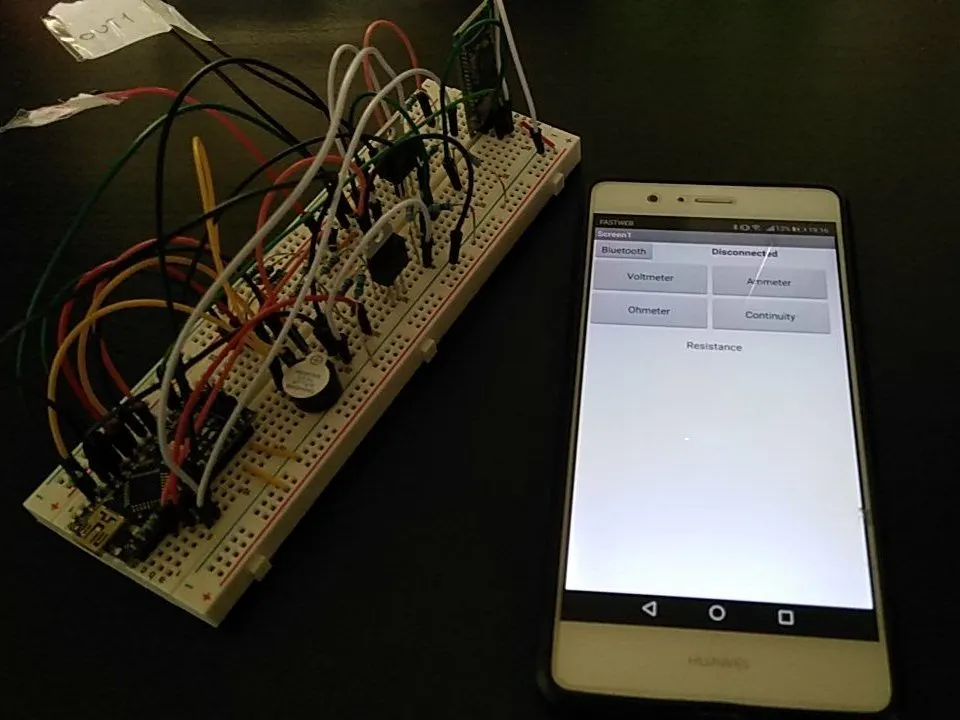A Bluetooth multimeter capable of resistance, voltage, current and continuity measurement. Made with Arduino Nano.

• 2,740 views
• 10 respects

## Components and suppliesArduino Nano R3
×1HC-06 Bluetooth Module
×1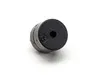Buzzer
×1Jumper wires (generic)
×1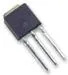Power MOSFET N-Channel
×2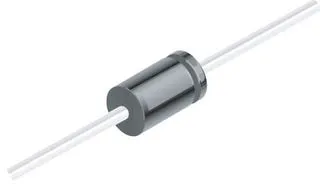1N4007 – High Voltage, High Current Rated Diode
×2Resistor 10k ohm
×1Resistor 1k ohm
×2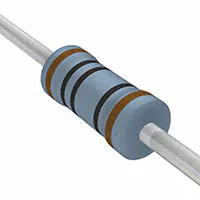Resistor 100 ohm
×1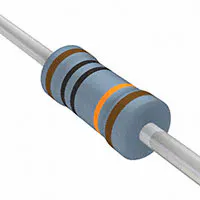Resistor 100k ohm
×1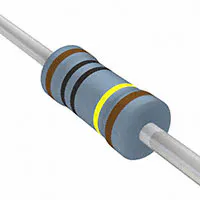Resistor 1M ohm
×3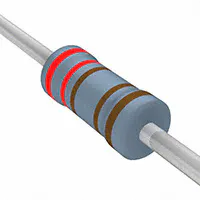Resistor 2.21k ohm
×1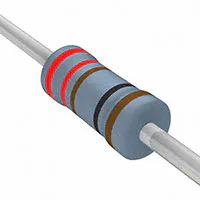Resistor 221 ohm
×2
 10 ohm resistor
×1

## Apps and online services

Hey everybody,

In this tutoria,l I'm going to show how to build a custom multimeter with Arduino called "Tinkerer Meter" capable of resistance/continuity/voltage/amperage measurement. And because I thought it would be fancy, the whole device can be controlled with an Android app via a Bluetooth module.

The tutorial will look through each part sequentially and will explain the code step by step. I hope you will enjoy the reading.

The first parts we are going to build is the ohmmeter and the Bluetooth module. Grab a bunch of resistor (100- 1k, 10k, 100k, 1M), a breadboard, your Arduino and a HC-06 module.

Figure 1 shows the wiring we are going to need for this to work.

Figure 1. Ohmmeter block and Bluetooth module wiring

For the Bluetooth (BT) Part the wiring is pretty simple. We connected the VCC and GND as always, while Arduino Rx is connected to BT TX. The connection Arduino Tx- BT-Rx requires instead a voltage divider made with a 1k and 2k ohm resistors in order to supply data to pin Rx at a maximum of 3.3V.

But how does a voltage divider work? This seems to be a silly question, but the answer to that will allow the building of almost every module of our multimeter.

Figure 2 shows a simple voltage divider as it can be found in every textbook.

Figure 2. A voltage divider

This circuit bring us to the most important relation we are going to use in this tutorial:

### Vout = Vi * (R2/(R1+R2))

In the case of the Bluetooth module, we know that Vin = 5V, and we require a Vout of 3.3V. If we use R1 = 1k and R2 = 2k:

Vout = 5 * (2/3) = 3.3 V

As we needed! Amazing, it works!

Lets try now another scenario. We still have Vin = 5 V and lets say that we are capable of measuring Vout using one of the analog pin of our Arduino. If we have one resistance value, this leave only one resistance as unknown. Solving the previous equation for that unknown resistance we can easily obtain that value.

In this way we can use the same logic we used to supply 3.3V to the BT module to build our Ohmmeter. The only thing to take care of is the fact that our error on the measurement of Vout ( needed for the unknown resistance calculation) gets wider and wider the more R1 and R2 (one of the two in unknown) are different. That explain why in the schematics are present a bunch of resistors ranging from 100 to 1Mohm. The trick to make this part of the device work comes from the Arduino code in figure 3.

``````[...]                      //NOTE: YOU CAN AVOID SAVING RESISTORS VALUE AS
int D2 = 2;                // VARIABLE, THIS WILL SAVE MEMORY. I DID THAT IN
int D3 = 3;                // ORDER TO CLARIFY HOW IT WORKS
int D4 = 4;
int D5 = 5;
int D6 = 6;
[...]
int R1 = 100;
int R2 = 1000;
int R3 = 10000;
long R4 = 100000;
long R5 = 1000000;
long Rn = 0;
[...]
int Analogohm = A0;
[...]
float raw = 0;
float Vout = 0;
float Ru = 0;
[...]

void loop() {
}
[...]
else if (measure == 'R'){
pinMode(D2, OUTPUT);
pinMode(D3, INPUT);
pinMode(D4, INPUT);
pinMode(D5, INPUT);
pinMode(D6, INPUT);
pinMode(D7, INPUT);
pinMode(D8, INPUT);
pinMode(D9, INPUT);
pinMode(D10, INPUT);
digitalWrite(D2, HIGH);
Rn = R5;
if (raw < 350 || raw > 800){
digitalWrite(D2, LOW);
pinMode(D2, INPUT);
pinMode(D3, OUTPUT);
digitalWrite(D3, HIGH);
Rn = R4;
if (raw < 350 || raw > 800){
digitalWrite(D3, LOW);
pinMode(D3, INPUT);
pinMode(D4, OUTPUT);
digitalWrite(D4, HIGH);
Rn = R3;
if (raw < 350 || raw > 800){
digitalWrite(D4, LOW);
pinMode(D4, INPUT);
pinMode(D5, OUTPUT);
digitalWrite(D5, HIGH);
Rn = R2;
if (raw < 350 || raw > 800){
digitalWrite(D5, LOW);
pinMode(D5, INPUT);
pinMode(D6, OUTPUT);
digitalWrite(D6, HIGH);
Rn = R1;
}
}
}
}
Vout = 5*(raw/1024);
Ru = (Rn*Vout)/(5-Vout);
Serial.print(Ru);
Serial.print(" Ohm");
delay(1000);
}
[...]
``````

Figure 3. Arduino code for the ohmmeter block

The first thing we need is declaring the variable needed: one digital output for each known resistor (and their value) and one analog input for voltage reading. Then in the void loop, the Arduino will read the value sent from the Bluetooth. If the ohmmeter behaviour is requested, the loop will enter the resistance calculation procedure as long as a new value is sent.

In this procedure, the first difference we can find from the usual Arduino code is that the pinMode is not defined in the void Setup, but it is defined inside the loop in order to change the pins behaviour. This is required because if we define all the digital pins as output without any change during the measurements, the pins will show low impedance even if we set them on OUTPUT LOW. What does this mean in the real world? It means that if you put one pin on HIGH in order to calculate the Vout on the voltage divider (example R1 - Ru series) the current will flow even in the other branch you thought it wouldn't because they were set on LOW! And this will obviously change the circuit behaviour.

But if we set the pins we don't use as digital INPUT, this guys will start showing really high impedance and thus no current will flow in their branch.

Once we get that the code in pretty easy to follow. It start from the branch with 1Mohm resistance and it measure Vout. The Arduino gives you back a value in the range [0-1024] with 1024 = 5V. Since we want to find the resistor whose value is close to the unknown, we must find the branch that give Vout = 512 = 2.5V. This thing can be explained using once again the voltage divider equation, if R1 = R2 we obtain Vout = Vin/2.

If Vout is lower (of higher) of a threshold value, the system moves to the next resistor, searching for the closest one.

In the final step it calculate the unknown resistance as explained before with the voltage divider equation solving for R2 (Ru in our example):

### Ru = (Rn * Vout) / ( Vin - Vout)

This value is then sent to a smartphone via the Serial.print() function.

The second block we are going to build is the continuity check. From the previous step you will need to add just few jumpers and an active buzzer (figure 4).

Figure 4. Schematics with the buzzer for continuity testing

Once again this module use the familiar voltage divider equation in order to check continuity in a cable. How does it work? You just need to touch the cable that need the continuity check and the pin D10 will supply 5V to the circuit. If the voltage read by the Analog Pin A4 is almost 0, then the continuity is fulfilled and the pin D9 will supply 5V to the buzzer in order to make noise.

This is summarized in the code in figure 5.

``````[...]
int D9 = 9;
int D10 = 10;
[...]
int Analogcontinuity = A4;
float raw = 0;
float Vout = 0;
float Ru = 0;
[...]

void loop() {
[...]
else if (measure == 'C'){
pinMode(D2, INPUT);
pinMode(D3, INPUT);
pinMode(D4, INPUT);
pinMode(D5, INPUT);
pinMode(D6, INPUT);
pinMode(D7, INPUT);
pinMode(D8, INPUT);
pinMode(D9, INPUT);
pinMode(D10, OUTPUT);
digitalWrite(D2, LOW);
digitalWrite(D3, LOW);
digitalWrite(D4, LOW);
digitalWrite(D5, LOW);
digitalWrite(D6, LOW);
digitalWrite(D7, LOW);
digitalWrite(D8, LOW);
digitalWrite(D9, LOW);
digitalWrite(D10, HIGH);
if (raw < 200){
pinMode(D9, OUTPUT);
digitalWrite(D9, HIGH);
Vout = 5*raw/1024;
Serial.print(Vout);
Serial.print(" V");
}
delay(1000);
}
``````

Figure 5. Code for the continuity block

Once again, if the right command is sent through the Bluetooth module, the loop will start the continuity statement and check out wire. As before every pin is set as INPUT to avoid any unwanted draw of current. Then if voltage read is below a threshold the buzzer will emit a sound and the voltage drop read will be sent through the serial port.

All the work done so far can be seen on a breadboard in the picture in figure 6.

Figure 6. Recap picture of the ohmmeter plus the continuity blocks

Moving to the next part, we are going to talk about the voltmeter. Lets take once again our voltage divider equation and lets assume this time that we know the value of the two resistances and, with the help of an Analog Pin, we are able to measure Vout. In this way we are able to calculate Vin. Here it comes the voltmeter. Since the maximum Vout we are able to measure is 5V, choosing the right combination of resistors is necessary to change the potential windows we are able to measure. Since we are tinkerer and we usually deal with low potentials, a series of 100k and 10k allow us to measure up to 55 V (you can verify this with the voltage divider equation). A n-mosfet is then used as a switch. Only if voltmeter mode is active this circuit will work, otherwise it is not connected to ground and no current will flow. In figure 7 are shown the wiring we need so far.

NOTE: The higher the resistances we use for the voltmeter, the lower the perturbation we make on the system we are measuring and thus the error.

Figure 7. Schematics with the voltmeter block

I highly suggest to put a diode in parallel with the mosfet, this will protect from parasite currents or if you plug the circuit you want to analyze in the wrong direction. I must be honest, I didn't use one of those because I was out of that, but really, you want to use that, the burnt mosfet is lurking for us all!

And the Arduino code is straight forward (figure 8).

``````[...]
int D7 = 7;
[...]
int AnalogVolt = A1;
[...]
float raw = 0;
float Vout = 0;
float Vin = 0;
[...]

void loop() {
}
if (measure == 'V') {
pinMode(D2, INPUT);
pinMode(D3, INPUT);
pinMode(D4, INPUT);
pinMode(D5, INPUT);
pinMode(D6, INPUT);
pinMode(D7, OUTPUT);
pinMode(D8, INPUT);
pinMode(D9, INPUT);
pinMode(D10, INPUT);
digitalWrite(D2, LOW);
digitalWrite(D3, LOW);
digitalWrite(D4, LOW);
digitalWrite(D5, LOW);
digitalWrite(D6, LOW);
digitalWrite(D7, HIGH);
digitalWrite(D8, LOW);
digitalWrite(D9, LOW);
digitalWrite(D10, LOW);
if(raw){
Vout = 5*raw/1024;
Vin = Vout * (R6 + R7)/R7;
Serial.print(Vin);
Serial.print(" V");
delay(1000);
}
``````

Figure 8. Arduino code for the voltmeter block

Just as we did before we define all pins as INPUT in order to exclude those from the circuit, the pin D7 is used to activate the mosfet and activate the voltmeter circuit. Vout is measured through analog pin A1 and Vin in computed as Vin = Vout * (R6 + R7)/ R7.

On a breadboard will result something like that in figure 9.

Figure 9. Ohmmeter plus continuity plus voltmeter blocks on a breadboard

The last thing we need to complete our Tinkerer Meter is an ammeter. This time we do not require the voltage divider equation, we just need Ohm's law.

Lets assume that we want to measure the current flowing in a circuit connected to a load. The easiest thing we can do is like the one in figure 10.

Figure 10. Current reroute for current measurements

We reroute the current from the target circuit through the low resistor in figure 10. Then by measuring the voltage before and after the resistor we can calculate the unknown current using the Ohm's law. Obviously this time we will need a resistor with really low value, such as all current may flow through our measuring branch, thus reducing the error.

Since one of the output we used for voltage/Resistance/Continuity measurement is grounded, we will need a different output this time to reconnect to the unknown circuit. Once again a mosfet is used as a switch for this branch to work.

Figure 11 and 12 show respectively the schematics and the code necessary.

Figure 11. Complete schematics of the device

``````[...]
int D8 = 8;
[...]
int AnalogAmmpost = A2;
int AnalogAmmpre = A3;
float raw = 0;
float raw2 = 0;
float Vout = 0;
[...]

void loop() {
}
else if (measure == 'A'){
pinMode(D2, INPUT);
pinMode(D3, INPUT);
pinMode(D4, INPUT);
pinMode(D5, INPUT);
pinMode(D6, INPUT);
pinMode(D7, INPUT);
pinMode(D8, OUTPUT);
pinMode(D9, INPUT);
pinMode(D10, INPUT);
digitalWrite(D2, LOW);
digitalWrite(D3, LOW);
digitalWrite(D4, LOW);
digitalWrite(D5, LOW);
digitalWrite(D6, LOW);
digitalWrite(D7, LOW);
digitalWrite(D8, HIGH);
digitalWrite(D9, LOW);
digitalWrite(D10, LOW);
Vout = 5*(raw - raw2)/1024;
current = Vout/R8;
Serial.print(current,4);
Serial.print(" A");
delay(1000);
}
``````

Figure 12. Arduino code for the ammeter block

This time the diode is even more necessary, if we invert the direction of the current we will burn it out for sure, so be sure to find one!

The code is just as those seen before, we set as output only pin D8 needed for mosfet activation, than the voltage pre- and post resistor is measured and the calculate current is printed, that 4 after the variable name in the Serial.print() tells that we want 4 digit instead of the default 2. This tells us also something else, we want to measure low current! High currents may burn your Arduino pins, watch out!

The result of our effort is in figure 13.

Figure 13. Full device on a Breadboard. Red cable IN, Black cable OUT1 (voltmeter and OUT2 (ammeter)

So far, so good, we have our device, now to fancy part, the Android app. You can easily write one with AppInventor2. Figure 14 shows the GUI prepared for our readings.

Figure 14. Android App GUI made with App Inventor 2

I know, it is really minimal, but I have a bad aestheticism.

More important for our purposes is the code, figure 15 shows the blocks used to build this app.

Figure 15. App Inventor code block

The code is easy to follow. After establishing the Bluetooth connection depending on the measure required data are sent to the Tinkerer Meter and the result are read on the smartphone screen.

ai2.appinventor.mit.edu/?galleryId=5701532933292032

In conclusion if you followed this tutorial so far you may have built your own digital multimeter with a Bluetooth interface. I hope you enjoyed this adventure as much I did. We all know that way more accurate multimeter can be bought for few euros out there, but now you know how to build one by yourself. This is more satisfying, isn't it?

Stay tuned for future laboratory equipment tutorial!

Feel free to ask question and report any error you may find!

## Code

##### Tinkerer Meter CodeArduino
This is the code for the tinkerer meter. After declaring the pin you are going to use, the void loop is divided into four if group that perform different calculation in order to calculate Resistance/Continuity/Amperage/Voltage depending of the value selected with the bluetooth app
```char measure = '0';
int D2 = 2;
int D3 = 3;
int D4 = 4;
int D5 = 5;
int D6 = 6;
int D7 = 7;
int D8 = 8;
int D9 = 9;
int D10 = 10;
int R1 = 100;
int R2 = 1000;
int R3 = 10000;
long R4 = 100000;
long R5 = 1000000;
long R6 = 100000;
int R7 = 10000;
int R8 = 10;
long Rn = 0;

int Analogohm = A0;
int AnalogVolt = A1;
int AnalogAmmpost = A2;
int AnalogAmmpre = A3;
int Analogcontinuity = A4;

float raw = 0;
float raw2 = 0;
float Vout = 0;
float Vin = 0;
float Ru = 0;
float current = 0;

void setup() {

Serial.begin(9600);

}

void loop() {

}

if (measure == 'V') {
pinMode(D2, INPUT);
pinMode(D3, INPUT);
pinMode(D4, INPUT);
pinMode(D5, INPUT);
pinMode(D6, INPUT);
pinMode(D7, OUTPUT);
pinMode(D8, INPUT);
pinMode(D9, INPUT);
pinMode(D10, INPUT);
digitalWrite(D2, LOW);
digitalWrite(D3, LOW);
digitalWrite(D4, LOW);
digitalWrite(D5, LOW);
digitalWrite(D6, LOW);
digitalWrite(D7, HIGH);
digitalWrite(D8, LOW);
digitalWrite(D9, LOW);
digitalWrite(D10, LOW);

if(raw){

Vout = 5*raw/1024;

Vin = Vout * (R6 + R7)/R7;

Serial.print(Vin);
Serial.print(" V");

delay(1000);
}
} else if (measure == 'R'){
pinMode(D2, OUTPUT);
pinMode(D3, INPUT);
pinMode(D4, INPUT);
pinMode(D5, INPUT);
pinMode(D6, INPUT);
pinMode(D7, INPUT);
pinMode(D8, INPUT);
pinMode(D9, INPUT);
pinMode(D10, INPUT);

digitalWrite(D2, HIGH);

Rn = R5;

if (raw < 350 || raw > 800){
digitalWrite(D2, LOW);
pinMode(D2, INPUT);
pinMode(D3, OUTPUT);
digitalWrite(D3, HIGH);
Rn = R4;

if (raw < 350 || raw > 800){
digitalWrite(D3, LOW);
pinMode(D3, INPUT);
pinMode(D4, OUTPUT);
digitalWrite(D4, HIGH);
Rn = R3;

if (raw < 350 || raw > 800){
digitalWrite(D4, LOW);
pinMode(D4, INPUT);
pinMode(D5, OUTPUT);
digitalWrite(D5, HIGH);
Rn = R2;

if (raw < 350 || raw > 800){
digitalWrite(D5, LOW);
pinMode(D5, INPUT);
pinMode(D6, OUTPUT);
digitalWrite(D6, HIGH);
Rn = R1;
}
}
}
}

Vout = 5*(raw/1024);
Ru = (Rn*Vout)/(5-Vout);
Serial.print(Ru);
Serial.print(" Ohm");
delay(1000);

} else if (measure == 'A'){
pinMode(D2, INPUT);
pinMode(D3, INPUT);
pinMode(D4, INPUT);
pinMode(D5, INPUT);
pinMode(D6, INPUT);
pinMode(D7, INPUT);
pinMode(D8, OUTPUT);
pinMode(D9, INPUT);
pinMode(D10, INPUT);
digitalWrite(D2, LOW);
digitalWrite(D3, LOW);
digitalWrite(D4, LOW);
digitalWrite(D5, LOW);
digitalWrite(D6, LOW);
digitalWrite(D7, LOW);
digitalWrite(D8, HIGH);
digitalWrite(D9, LOW);
digitalWrite(D10, LOW);

Vout = 5*(raw - raw2)/1024;
current = Vout/R8;
Serial.print(current,4);
Serial.print(" A");
delay(1000);

} else if (measure == 'C'){
pinMode(D2, INPUT);
pinMode(D3, INPUT);
pinMode(D4, INPUT);
pinMode(D5, INPUT);
pinMode(D6, INPUT);
pinMode(D7, INPUT);
pinMode(D8, INPUT);
pinMode(D9, INPUT);
pinMode(D10, OUTPUT);
digitalWrite(D2, LOW);
digitalWrite(D3, LOW);
digitalWrite(D4, LOW);
digitalWrite(D5, LOW);
digitalWrite(D6, LOW);
digitalWrite(D7, LOW);
digitalWrite(D8, LOW);
digitalWrite(D9, LOW);
digitalWrite(D10, HIGH);
if (raw < 200){
pinMode(D9, OUTPUT);
digitalWrite(D9, HIGH);
Vout = 5*raw/1024;
Serial.print(Vout);
Serial.print(" V");
}
delay(1000);
}

}
```

## Schematics

As for the code, this schematic can be divided into four block (one for each function) plus the Bluetooth module. I suggest to follow the tutorial for the explanation of each component.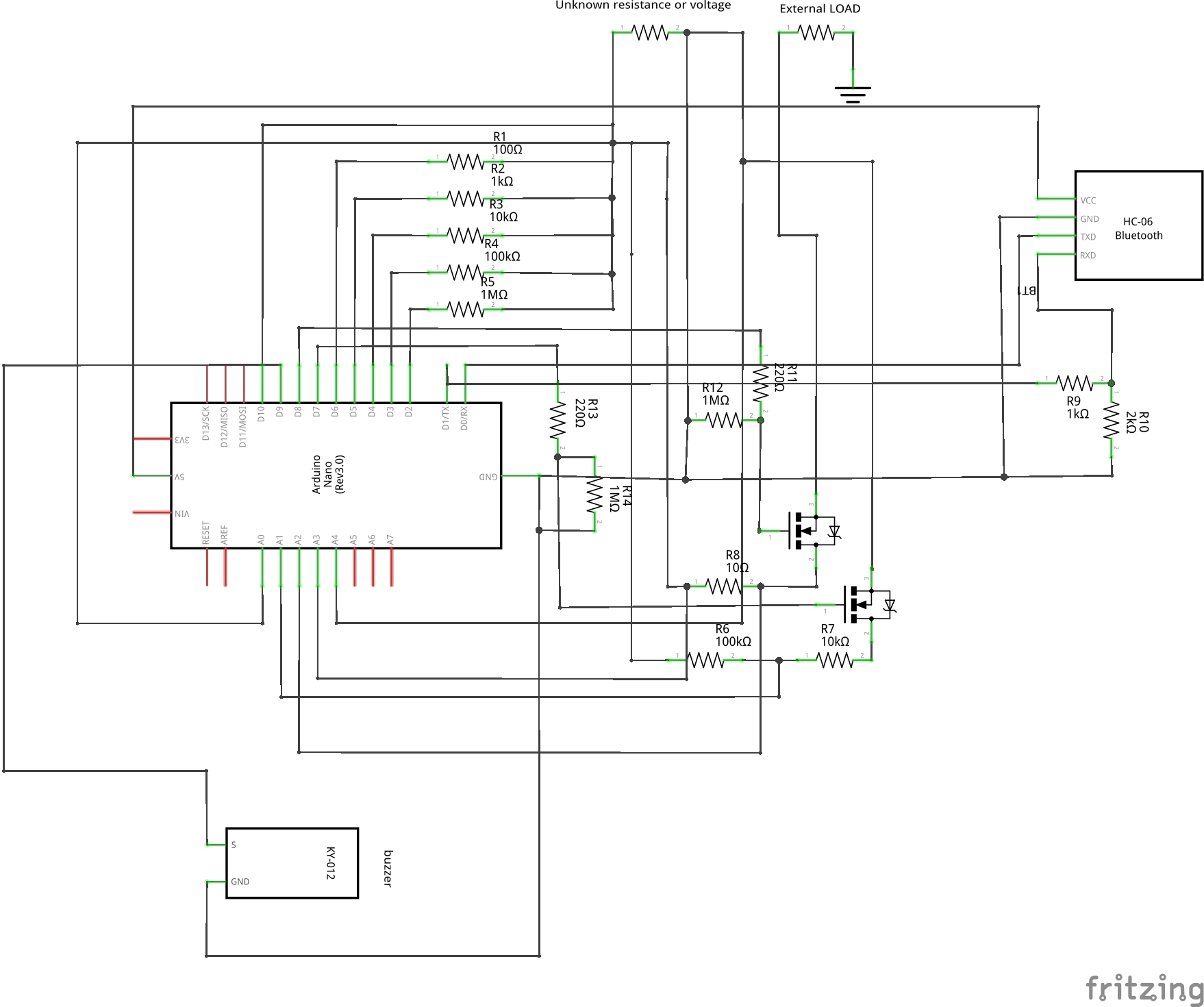This is a variation of the basic schematic with a protecting diode for each transistor. I didn't do that because I was out of those, but I highly suggest to follow this variation, since it would prevent the destruction of the transistor if you connect the pin in the wrong way.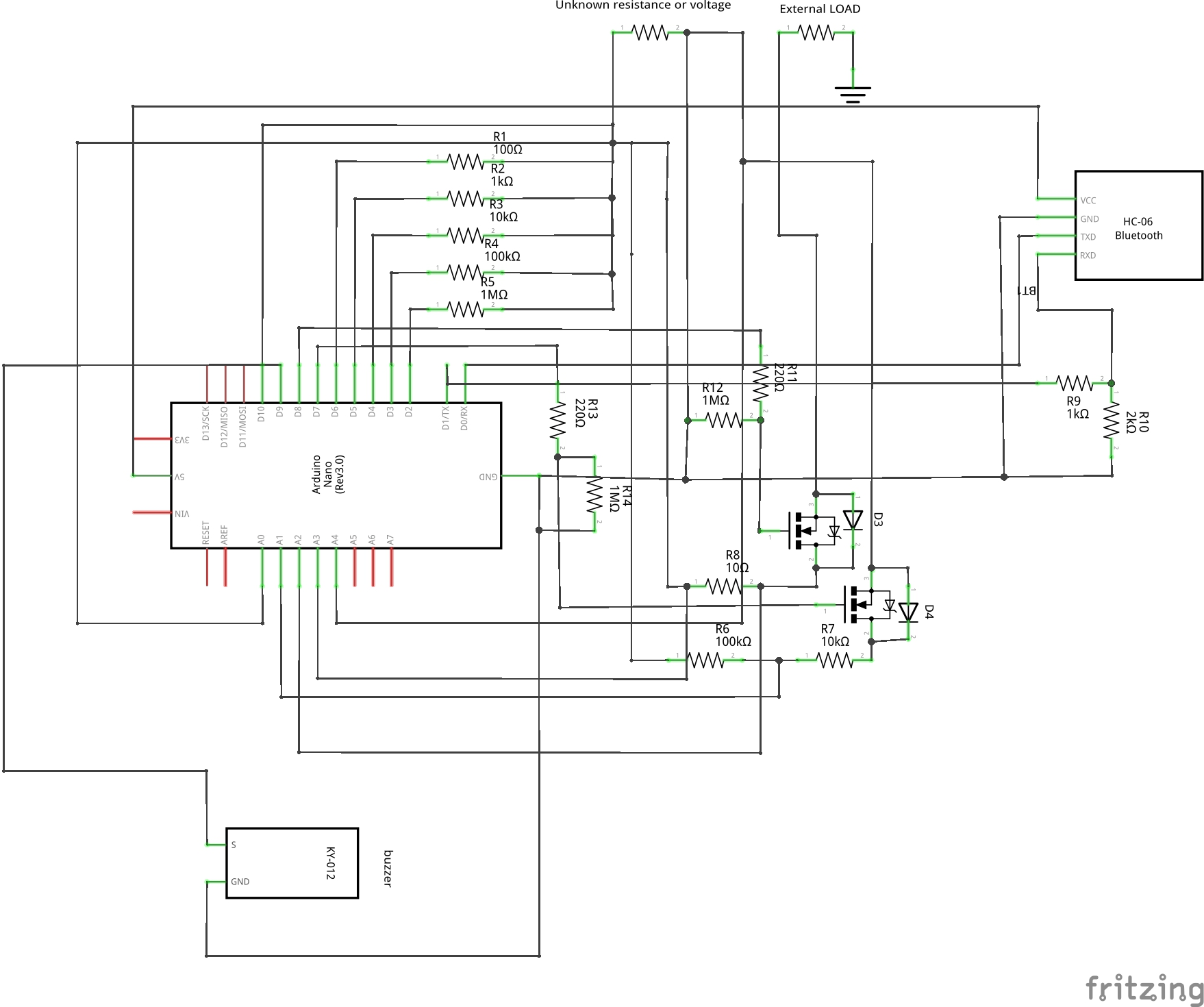#### Author• 4 projects
• 22 followers

#### Published on

June 9, 2019
See similar projects
you might like

#### Bluetooth Temperature Monitor

Project tutorial by Federico Vivaldi

• 4,935 views
• 15 respects

#### Sorting Resistors the Lazy Way

Project tutorial by Federico Vivaldi

• 12,389 views
• 82 respects

#### Mobile Water Level Tracker

Project tutorial by Kutluhan Aktar

• 3,478 views
• 6 respects

#### Sigfox kWh Meter

Project tutorial by jassak

• 7,509 views
• 15 respects

#### Create a People Counter Controlled by an Android App

Project tutorial by Kutluhan Aktar

• 7,383 views